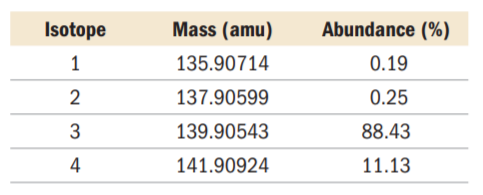×
Get Full Access to Chemistry: A Molecular Approach - 3 Edition - Chapter 2 - Problem 76e
Get Full Access to Chemistry: A Molecular Approach - 3 Edition - Chapter 2 - Problem 76e

×

# An element has four naturally occurring isotopes with theISBN: 9780321809247 1

## Solution for problem 76E Chapter 2

Chemistry: A Molecular Approach | 3rd Edition

• Textbook Solutions
• 2901 Step-by-step solutions solved by professors and subject experts
• Get 24/7 help from StudySoup virtual teaching assistantsChemistry: A Molecular Approach | 3rd Edition

4 5 1 292 Reviews
17
1
Problem 76E

An element has four naturally occurring isotopes with the masses and natural abundances given here. Find the atomic mass of the element and identify it.Step-by-Step Solution:

Solution : Step 1: In this problem, we are supposed to give the name or chemical formulas for the given acids. So let’s see what is an acid and then we will look into rules for naming acids and for writing chemical formulas and then we would write the formulas and name depending upon the compounds given.

Step 2 of 3

Step 3 of 3

##### ISBN: 9780321809247

Since the solution to 76E from 2 chapter was answered, more than 561 students have viewed the full step-by-step answer. This full solution covers the following key subjects: acid, compounds, inorganic, chemical, chloric. This expansive textbook survival guide covers 82 chapters, and 9454 solutions. The answer to “?An element has four naturally occurring isotopes with the masses and natural abundances given here. Find the atomic mass of the element and identify it.” is broken down into a number of easy to follow steps, and 25 words. Chemistry: A Molecular Approach was written by and is associated to the ISBN: 9780321809247. The full step-by-step solution to problem: 76E from chapter: 2 was answered by , our top Chemistry solution expert on 02/22/17, 04:35PM. This textbook survival guide was created for the textbook: Chemistry: A Molecular Approach, edition: 3.

## Discover and learn what students are asking

Chemistry: The Molecular Nature of Matter and Change : Kinetics: Rates and Mechanisms of Chemical Reactions
Assume water boils at 100.0?C in Houston (near sea level), and at 90.0?C in Cripple Creek, Colorado (near 9500 ft). If it takes 4.8 min to cook an egg

Statistics: Informed Decisions Using Data : Comparing Three or More Means (One-Way Analysis of Variance)
?True or False: To perform a one-way ANOVA, the populations do not need to be normally distributed.

Unlock Textbook Solution